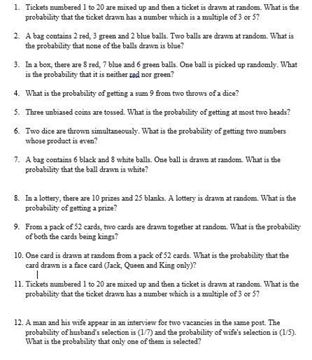# Revision Notes For CBSE Class 9 Math Chapter -15 Probability

Probability is a branch of maths which deals with calculating the likelihood of an event happening. Directly or indirectly, probability plays an important role in day to day activities.

For example, Rolling a die or flipping a coin. When we flip a coin, there are only two possible outcomes, the result is either heads or tail. In the same way, when we roll a die, there are six possible outcomes, the result may be any of these- 1, 2, 3, 4, 5, 6.

The formula for calculating the probability is:

Probability = Number of favourable outcomes / Total number of possible outcomes

For Example

There are 6 fruits in a bowl, 3 are apple, 2 are guava and 1 is banana. What is the probability of picking a guava?

The probability is the number of guava in the bag divided by the total number of fruits = P(A) = NO of favourable outcomes / Total number of possible outcomes

= P(A) = 2 / 6 = 1 / 3.

Check here for the free CBSE Class 9 Math revision notes for Chapter 15- Probability.